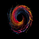# Ehlers Adaptive Cyber Cycle Indicator [LazyBear]

9367 obejrzeń
9367
Another famous Ehlers indicator.

This is the adaptive version of Ehlers' Cyber Cycle ( CC ) (already published, check "More info" below). Idea behind making something "adaptive" is to calculate it using dynamic cycle period inputs instead of static setting. In adaptive cyber cycle, Ehlers uses the dominant cycle period as the length in computation of alpha.

According to Ehlers this should be more responsive than the non-adaptive version. Buy and sell signals should often occur one bar earlier than for the non-adaptive version.

I have the usual options in place. Check out plain CC for comparison.

- Cyber Cycle Indicator: - Cybernetic Analysis for Stocks and Futures ( Ehlers )

List of my public indicators: http://bit.ly/1LQaPK8
List of my app-store indicators: http://blog.tradingview.com/?p=970
```//
// @author LazyBear
//
// List of my public indicators: http://bit.ly/1LQaPK8
// List of my app-store indicators: http://blog.tradingview.com/?p=970
//
study("Ehlers Adaptive Cyber Cycle Indicator [LazyBear]", shorttitle="EACCI_LB", overlay=false, precision=3)
src=input(hl2, title="Source")
a=input(.07, title="Alpha")
s = (src + 2*src + 2*src + src)/6.0
c = n<7?(src - 2*src + src)/4.0:((1 - 0.5*a)*(1 - 0.5*a)*(s - 2*s + s) + 2*(1-a)*c - (1 - a)*(1-a)*c)
q1 = (.0962*c + 0.5769*c - 0.5769*c - .0962*c)*(0.5+.08*nz(ip))
I1 = c
dp_ = iff(q1 != 0 and q1 != 0, (I1/q1 - I1/q1) / (1 + I1*I1/(q1*q1)),0)
dp = iff(dp_ < 0.1, 0.1, iff(dp_ > 1.1, 1.1, dp_))
med(x,y,z) => (x+y+z) - min(x,min(y,z)) - max(x,max(y,z))
md = med(dp,dp, med(dp, dp, dp))
dc = iff(md == 0, 15, 6.28318 / md + 0.5)
ip = .33*dc + .67*nz(ip)
p = .15*ip + .85*nz(p)
a1 = 2.0/(p + 1)
ac=nz(((1-0.5*a1)*(1-0.5*a)*(s-2*s+s)+2*(1-a1)*ac-(1-a1)*(1-a1)*ac), (src-2*src+src)/4.0)
t=ac
fr=input(true, title="Fill Osc/Trigger region")
plot(0, color=gray, title="ZeroLine")
duml=plot(fr?(ac>t?ac:t):na, style=circles, linewidth=0, color=gray, title="Dummy")
tl=plot(t, title="Trigger",color=green)
fill(cmil, duml, color=red, transp=50, title="NegativeFill")
fill(tl, duml, color=lime, transp=50, title="PositiveFill")
ebc=input(false, title="Color bars?")
bc=ebc?(ac>0? (ac>t?lime:(ac==t?gray:green)): (ac<t?red:orange)):na
barcolor(bc)
```could you please elaborate on the parameter "ip" in the code?

it is not defined in the code prior to being implemented in the calculation of "q1".

I am using a different language to Easylanguage and get a formatting error "undefined or unexpected character"

I am new to coding financial indicators (as you have probably guessed) and would very much appreciate any help.

thank you
Odpowiedźhillab
@hillab, The problem seems to be circular dependencies in the code. The variable ip depends on another variable which depends on another variable which depends on ip. This was not a problem in earlier versions of easylanguage because the implementation of time was more sloppy - the scripts in version 2 could see into the future, called repainting - but this has fortunately been adressed in version 3. The downside is that a lot of the older script need to be reworked. In this case I would say that it is a good thing since it is quite messy code even if the indicator itself is useful.
Odpowiedźmjp152
This version below is updated for use in Easylanguage version 3 - the circular dependencies are handled by assigning na-value series to the involved variables and then reassigning actual values to them as the calculations are made (using the ":=" operator). Hope this is useful.

//@version=3
//
// @author LazyBear
//
// List of my public indicators: http://bit.ly/1LQaPK8
// List of my app-store indicators: http://blog.tradingview.com/?p=970
//
study("Ehlers Adaptive Cyber Cycle Indicator ", shorttitle="EACCI_LB", overlay=false, precision=3)

// Inputs
src=input(hl2, title="Source")
a=input(.07, title="Alpha")
fr=input(true, title="Fill Osc/Trigger region")

c = na
ip = na
p = na
ac = na

s = (src + 2*src + 2*src + src)/6.0
c := n<7?(src - 2*src + src)/4.0:((1 - 0.5*a)*(1 - 0.5*a)*(s - 2*s + s) + 2*(1-a)*c - (1 - a)*(1-a)*c)
q1 = (.0962*c + 0.5769*c - 0.5769*c - .0962*c)*(0.5+.08*nz(ip))
I1 = c
dp_ = iff(q1 != 0 and q1 != 0, (I1/q1 - I1/q1) / (1 + I1*I1/(q1*q1)),0)
dp = iff(dp_ < 0.1, 0.1, iff(dp_ > 1.1, 1.1, dp_))
med(x,y,z) => (x+y+z) - min(x,min(y,z)) - max(x,max(y,z))
md = med(dp,dp, med(dp, dp, dp))
dc = iff(md == 0, 15, 6.28318 / md + 0.5)
ip := .33*dc + .67*nz(ip)
p := .15*ip + .85*nz(p)
a1 = 2.0/(p + 1)
ac := nz(((1-0.5*a1)*(1-0.5*a)*(s-2*s+s)+2*(1-a1)*ac-(1-a1)*(1-a1)*ac), (src-2*src+src)/4.0)
t = ac

// Plotting
plot(0, color=gray, title="ZeroLine")
duml=plot(fr?(ac>t?ac:t):na, style=circles, linewidth=0, color=gray, title="Dummy")
tl=plot(t, title="Trigger",color=green)
fill(cmil, duml, color=red, transp=50, title="NegativeFill")
fill(tl, duml, color=lime, transp=50, title="PositiveFill")
ebc=input(false, title="Color bars?")
bc=ebc?(ac>0? (ac>t?lime:(ac==t?gray:green)): (ac<t?red:orange)):na
barcolor(bc)
OdpowiedźSince you already coded so many Ehlers indicators this week I was afraid to ask for even more Ehlers :) I'm happy you decided yourself to complete the collection with the adaptive versions. In my test it surprised me that the Adaptive Cyber Cycle seems to be faster than the Adaptive Center Of Gravity (CG) indicator. I expected the Center Of Gravity to be faster, because it behaves similar to the classic stochastic indicator (which is very fast).

OdpowiedźAnother interesting early indication from ACC.

Odpowiedź